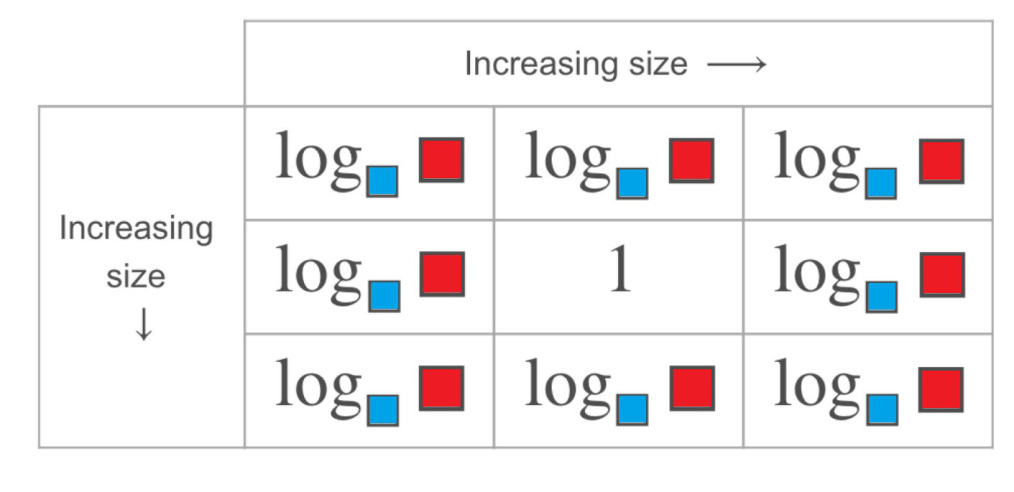# Logs

Directions: Using the integers 1 to 9, fill in the red and blue boxes so that the chart is accurate. You can only use a number once per red box and once per blue box.### Hint

What mathematical equation can be produced from a logarithm? How does that relate to the size of a logarithm?

### Answer

Source: Bryan Anderson

## Creating Sequences

Directions: Using the digits 0-9, at most one time each, complete the first three terms …